Transforming Variables

# Common Transformations

The most common transformations are available in the Edit:Variables menu. For example, log transformations are commonly used to linearize relationships, stabilize variances, or reduce skewness. Perform a log transformation in a fit window by following these steps: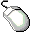Open the BASEBALL data set.Create a fit analysis of SALARY versus CR_HOME.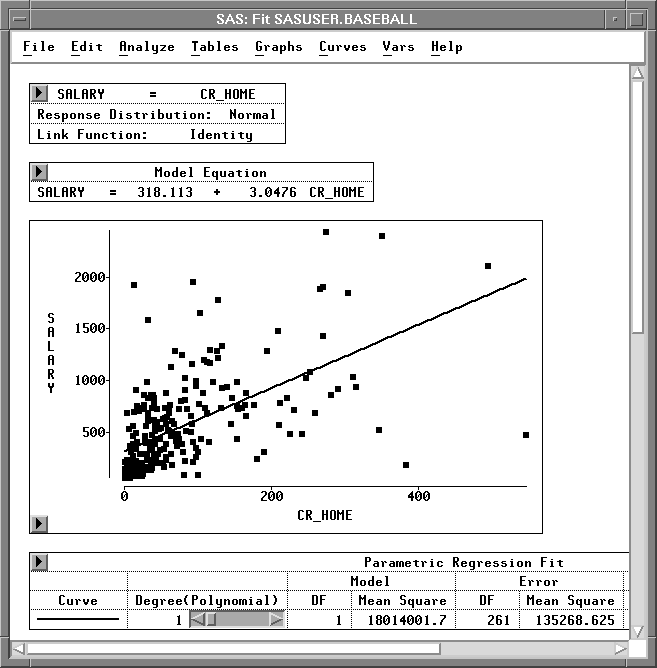Figure 20.2: Fit Analysis of SALARY versus CR_HOME

You might expect players who hit many home runs to receive high salaries. However, most players do not hit many home runs, and most do not have high salaries. This obscures the relationship between SALARY and CR_HOME. Most of the observations appear in the lower left corner of the scatter plot, and the regression line does not fit the data well. To make the relationship clearer, apply a logarithmic transformation.Select both variables in the scatter plot.

Use your host's method for noncontiguous selection.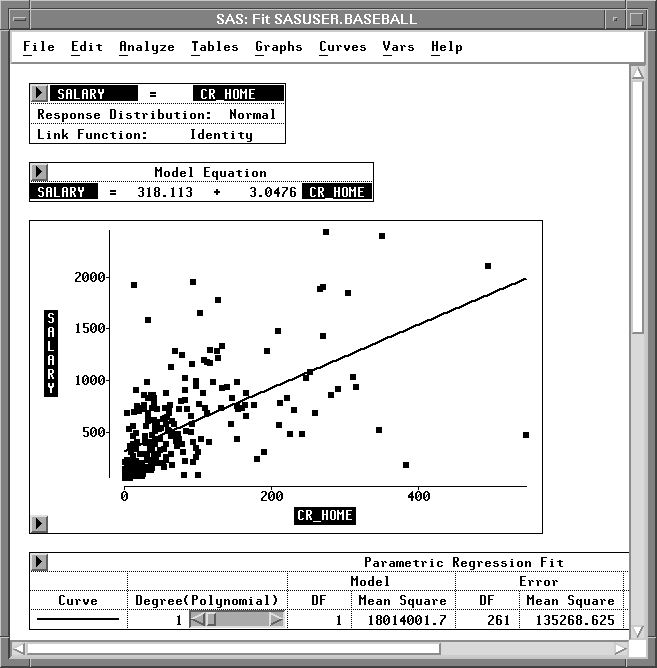Figure 20.3: SALARY and CR_HOME SelectedChoose Edit:Variables:log(Y).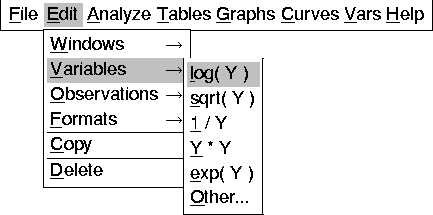Figure 20.4: Edit:Variables Menu

This performs a log transformation on both SALARY and CR_HOME and transforms the scatter plot to a log-log plot. Now the regression fit is improved, and the relationship between salary and home run production is clearer.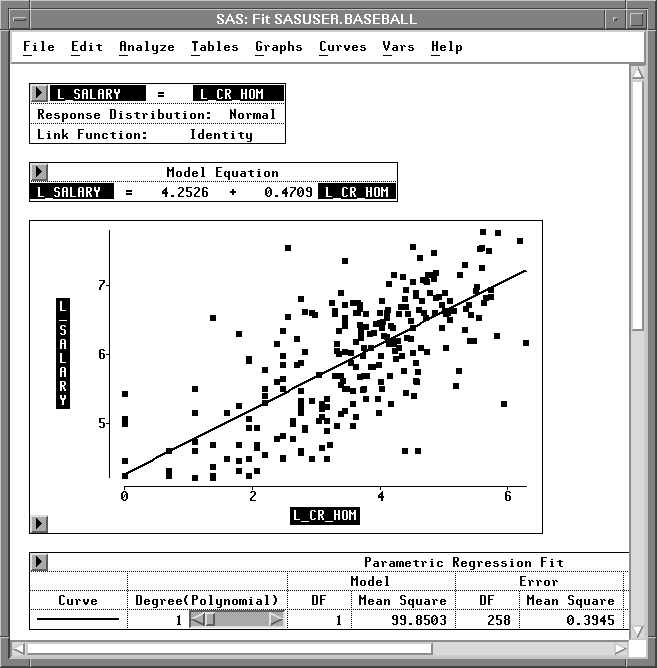Figure 20.5: Fit Analysis of L_SALARY versus L_CR_HOM

The degrees of freedom (DF) is reduced from 261 to 258. This is due to missing values resulting from the log transformation, described in the following step.Scroll the data window to display the last four variables.

Notice that in addition to residual and predicted values from the regression, the log transformations created two new variables: L_SALARY and L_CR_HOM.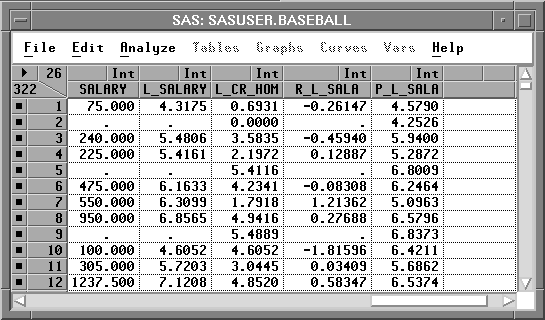Figure 20.6: New Variables

The log transformation is useful in many cases. However, the result of log( Y ) is undefined where Y is less than or equal to 0. In such cases, SAS/INSIGHT software cannot transform the value, so a missing value (.) is generated. To see this, sort the data in the data window.Select L_CR_HOM in the data window, and choose Sort from the data pop-up menu.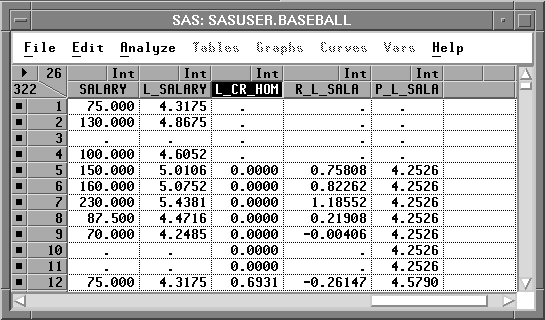Figure 20.7: Missing Values in Log Transformation

Missing values in the SAS System are considered to be less than any other value, so they appear first in the sorted variable. These values represent players who have never hit home runs. Their value for CR_HOME is 0, so the log of this value cannot be calculated. This means the log transformation has removed data from the fit analysis. The following steps circumvent this problem.Select CR_HOME in the data window.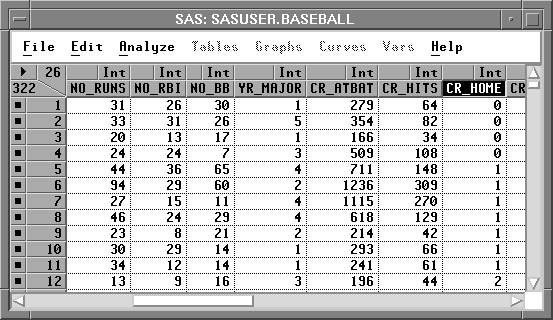Figure 20.8: CR_HOME SelectedChoose Edit:Variables:Other.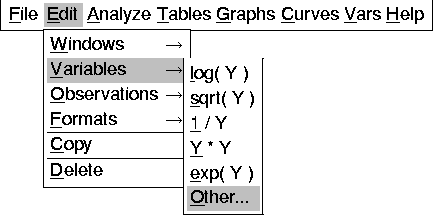Figure 20.9: Edit:Variables Menu

This displays the Edit Variables dialog shown in Figure 20.10. In the dialog you can see that the variable CR_HOME is already assigned as the Y variable.Scroll down the transformation window, and select log( Y + a ).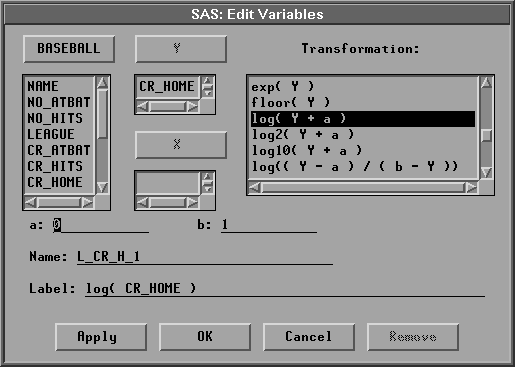Figure 20.10: Edit Variables DialogIn the field for a enter the value 1, then press the Return key.

Notice that the Label value changes from log( CR_HOME ) to log( CR_HOME + 1 ) to reflect the new value of a. Setting a to 1 avoids the problem of generating missing values because (CR_HOME + 1) is greater than zero in all cases for this data.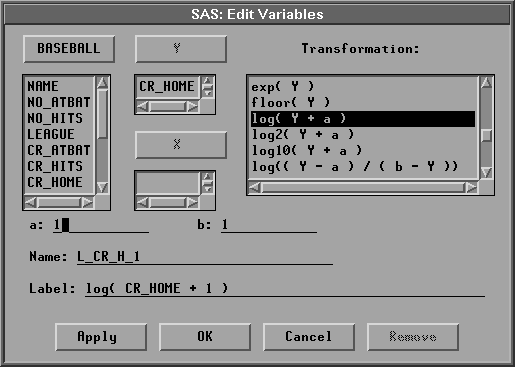Figure 20.11: Edit Variables DialogClick OK to perform the transformation.Scroll all the way to the right to see the new variable, L_CR_H_1.

Notice that the new variable contains no missing values.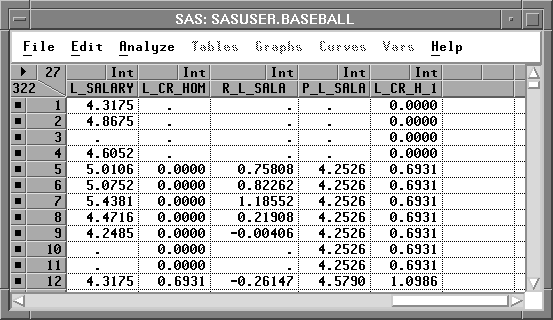Figure 20.12: New VariableSelect L_SALARY and L_CR_H_1, then choose Analyze:Fit (Y X).

At the lower left corner of the scatter plot, you can see observations that were not used in the previous fit analysis. Also note that the degrees of freedom (DF) is back to 261.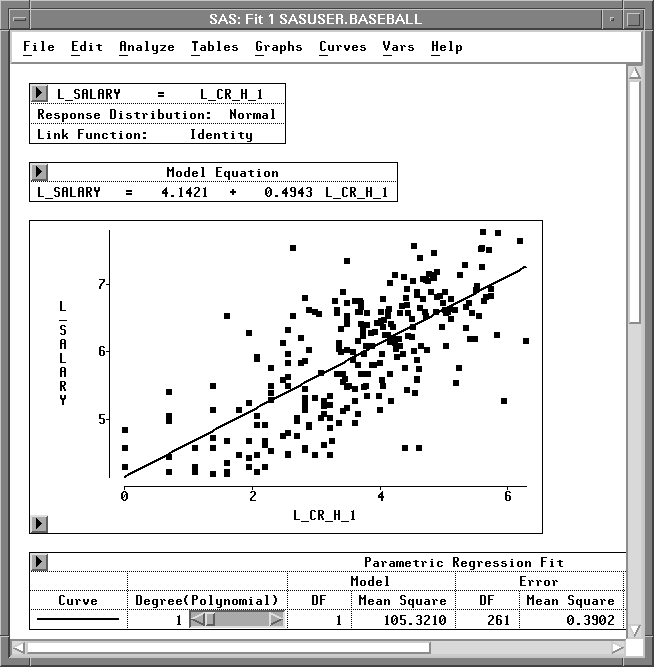Figure 20.13: New Fit Analysis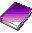Related Reading Linear Models, Chapter 39.

Copyright © 2007 by SAS Institute Inc., Cary, NC, USA. All rights reserved.